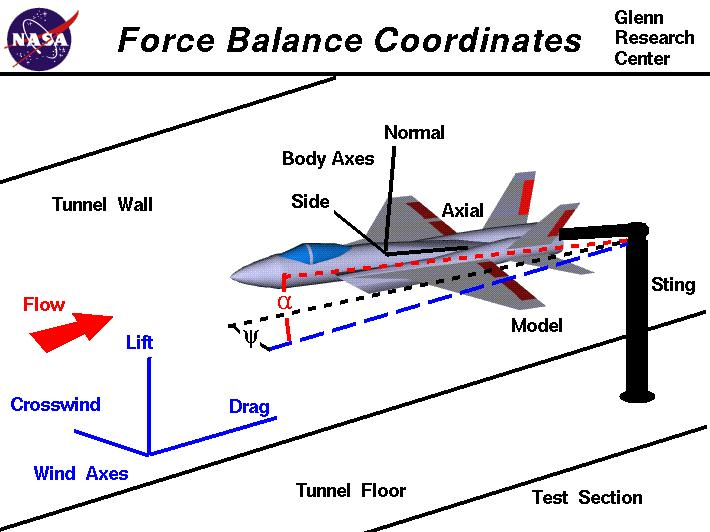+ Text Only Site
+ Non-Flash Version
+ Contact GlennAerodynamicists use wind tunnels to test models of proposed aircraft and engine components. During a test, the model is placed in the test section of the tunnel and air is made to flow past the model. Various types of tests can be run in a wind tunnel. Some tests are performed to directly measure the aerodynamic forces and moments on the model. The most basic type of instrument used in this type of testing is the force balance. We live in a world that is described by three, mutually perpendicular, spatial dimensions; left to right, up and down, back and forth. To completely describe the conditions on the aircaft model, we must therefore measure the forces in each of three directions and the moments, or rotations, about three directions. There are several different coordinate systems that can be used to describe three-dimensional space. On the figure we show two such coordinate systems, the wind axes which are aligned with walls of the wind tunnel, and the body axes which are aligned to the geometry of the aircraft model. If the force balance is placed inside the model, it measures forces and moments along the body axes. If the force balance is external to the model, it measures forces and moments along the wind axes. The type of model mount employed can also limit the types of measurements that can be made and the axes of the measurements. Whether we use an internal balance or an external balance, we must eventually resolve the measured forces into lift, drag, and side force, and the moments into pitching moment, rolling moment, and yawing moment. Drag and lift are defined to be the components of the aerodynamic force in the streamwise and perpendicular flow directions respectively. So lift, drag and side force are directly measured in the wind axes. To bring the measurements in the body axes into the wind axes requires some trigonometry. On the figure, the model is inclined to the wind axes by two angles. Alpha (the angle in red) is the angle of attack, and psi (the angle in black) is the angle of yaw. We can relate the measurements in the body axes to the wind axes by the following equations. Let's let A = the measured axial force, N be the measured normal force, S be the measured side force in the body axes. Similarly let AM be the moment about the axial axis, NM be the moment about the normal axis, and SM be the moment about the side aixs. The lift L is given by: L = N cos(alpha) - A sin(alpha) The drag D is given by: D = A cos(alpha) cos(psi) - N sin(alpha) cos(psi) The crosswind force C is: C = S cos(psi) + A cos(alpha) sin(psi) - N sin(alpha) sin(psi) Converting the moments is a little more difficult than the forces because the moments are defined about a certain point on the aircraft. With b as the wing span and c as the mean aerodynamic chord, the pitching moment PM is given by PM = SM cos(psi) + (b/c)AM cos(alpha)sin(psi) + (b/c)NM sin(alpha) sin(psi) Similarly, the yawing moment (YM) is equal to YM = NM cos(alpha) - AM sin(alpha) and the rolling moment (RM) is equal to RM = AM cos(alpha)cos(psi) - (c/b)SM sin(psi) + NM sin(alpha)sin(psi) Navigation ..Beginner's Guide Home Page+ Inspector General Hotline + Equal Employment Opportunity Data Posted Pursuant to the No Fear Act + Budgets, Strategic Plans and Accountability Reports + Freedom of Information Act + The President's Management Agenda + NASA Privacy Statement, Disclaimer, and Accessibility CertificationEditor: Nancy Hall NASA Official: Nancy Hall Last Updated: May 13 2021 + Contact Glenn